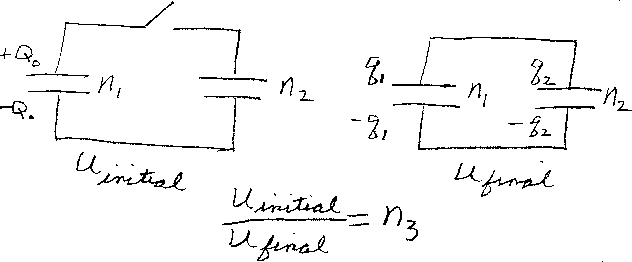Problem B10: Consider the two capacitors shown in the figure above. The left one has a capacitance of n1 Farads, and the right one has a capacitance of n2 Farads. Initially, the left capacitor is charged and the right capacitor is uncharged. After the switch is closed, charge flows from the left to the right capacitor. After the system comes to equilibrium, what is the ratio of the initial energy, Uinitial, to the final energy Ufinal? That is, if Uinitial/Ufinal = n3, what is n3?n1 = n2 = Input n3:
If you are currently in my class, you can record your grade by entering your name and student ID number (without the leading zeros) below and clicking on "record grade".
 First Name = Last Name = ID = Problem: